Algebra 2 Introduction, Basic Review, Factoring, Slope, Absolute Value, Linear, Quadratic EquationsAlgebra 2 Introduction, Basic Review, Factoring, Slope, Absolute Value, Linear, Quadratic Equations

This algebra 2 introduction / basic review lesson video tutorial covers topics such as solving linear equations, absolute value equations, inequalities, and quadratic equations. It shows you how to factor trinomials and polynomials in addition to simplify rational and radical expressions. This video contains a ton of examples and practice problems.

My Website:
https://www.video-tutor.net

Need Help With Difficult Homework Problems?
https://bit.ly/Find-A-Tutor

Patreon: https://www.patreon.com/MathScienceTutor
Amazon Store: https://www.amazon.com/shop/theorganicchemistrytutor

Algebra Video Playlist:

https://amzn.to/2RYDGLO

HP Envy X2 15 Laptop: (This was my old laptop)
https://amzn.to/3xswE2o

Microsoft Suface Book:
https://amzn.to/3nAYkxz

Intuos Wacom Table:
https://amzn.to/3vnVwpW

Here is a list of topics:
1. Algebra 2 Introduction – Basic Review lesson
2. Solving Linear Equations With Variables, Parentheses and Fractions
3. Solving and Graphing Inequalities on a Number line
4 Solving Absolute Value Equations With Inequalities
5. Calculating the Slope Between Two Points With Fractions
6. Writing Linear Equations In Slope Intercept Form, Point Slope Form, and Standard Form
7. Parallel and Perpendicular Lines
8. Rate of Change Problems – Formula & Calculations
9. Graphing Linear Equations Using the Slope and Y Intercept
10. Graphing Linear Equations Using X and Y Intercepts
11. Horizontal and Vertical Lines
12. Graphing Absolute Value Equations
13. Parent Functions and Transformations
14. Systems of Equations – Substitution, Elimination and Graphically – Point of Intersection
15. Horizontal and Vertical Shifts – Reflection about X and Y axis
16. Factoring Binomials – Difference of Perfect Squares Method
17. Factoring Trinomials – Leading Coefficient
18. Factoring By Grouping and GCF Method
19. Factoring Polynomials – Cubic and With Synthetic Division
20. Solving Quadratic Equations By Factoring
21. Solving Quadratic Equations By Completing The Square
23. Graphing Quadratic Equations In Standard and Vertex Form
24. Vertex, Axis of Symmetry, Maximum and Minimum Values
25. Domain and Range of Linear, Absolute Value and Quadratic Equations – Interval Notation
26. Discriminant Formula – Real Solutions vs Imaginary Solutions
27. Imaginary Numbers – Adding, Subtracting, Multiplying, Dividing and Simplifying
28. Graphing Complex Numbers – Absolute Value Calculation
29. Exponent Properties – Multiplying and Dividing
30. Negative Exponents – Simplifying
31. Zeros of Polynomial Functions
32. List of Possible Rational Zeros
33. Synthetic Division and Long Division
34. Factoring Cubic Polynomials Using Synthetic Division and Factoring By Grouping
35. Evaluating Functions Using Synthetic Division
36. Inverse Functions
37. Composite Functions – F(g(x))
38. Evaluating Logarithmic Functions
39. Properties of Logarithms
40. Condensing, Expanding and Solving Logarithmic Equations
42. Graphing Square Root / Radical Equations With Transformation – Domain and Range
43. Simplifying Rational Expressions and Solving Rational Equations
44. Rational Expressions – Adding, Subtracting, Multiplying and Dividing
45. Rationalizing the Denominator
46. Graphing Rational Equations
47. Horizontal and Vertical Asymptotes Plus Holes

algebra tutors,algebra 2,introduction,algebra 2 review,basics,factoring,lessons,completing the square,slope,quadratic equations,absolute value equations,algebra,factoring polynomials,slope intercept form,domain,inequalities,range,logarithms,linear equations,exponents,solving,graphing,quadratic formula,functions,system of equations,imaginary numbers,inverse,complex numbers,composite functions,asymptotes,parent functions,transformations,parallel and perpendicular lines

algebratutors.org

48 Responses to “Algebra 2 Introduction, Basic Review, Factoring, Slope, Absolute Value, Linear, Quadratic Equations”

1.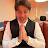Anthony Fuentes says:

Also if your here because of your finals just know you got this and the effort you are putting by watching this video will guarantee your success… compared to if you did nothing… you are doing great 👍

2.Anthony Fuentes says:

If I sleep while listening to this I will subconsciously understand all the material for my final on Wednesday 😌

3.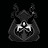Deciphered Void says:

Why the hell did I get this in my recommended?

4.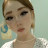天使 says:

the fact i am 13 and already taking algebra 2 and calculus makes me feel like einstein.

5.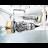Adesoji Adegite says:

https://youtu.be/MgDKj74mBs8

6.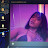j4yla says:

bro i love you

7.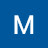Magdy Hussein says:

Even though I teach math, I always enjoy watching your great videos.

8.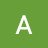Dodge3031 Maskcat says:

isn't this exactly what you taught in the algebra 1 video?

9.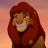Corey Barton says:

30:00

10.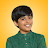Reeko Not rookie says:

1:38:40

11.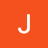Not Jenna says:

All I did was combine the top comment and the other timestamps. Have fun studying with me lol
📏 LINEAR EQUATIONS 📏

0:07 | Basic

1:03 | Variables on Both Sides

2:24 | Parentheses/Distribution

5:00 | Fraction Coefficients

6:06 | Fraction Coefficients and Constants

7:39 | Fractions on Both Sides (Cross Multiplication)

📐 INEQUALITIES 📐

8:31 | Basic Graphing

10:36 | Interval Notation

11:33 | Inequality Equations

13:45 | OR Inequalities

15:53 | AND Inequalities

📘 ABSOLUTE VALUE 📘

17:40 | Basic

18:17 | Equations

20:30 | Equations with Numbers Outside

22:50 | Absolute Value Inequalities

26:38 | Inequalities with Numbers Outside

📈 GRAPHING 📈

30:04 | Basic Slope-Intercept

31:54 | Fraction Slope

33:07 | Standard Form

35:21 | Linear Inequalities

41:48 | Absolute Value Functions

49:50 | Absolute Value Inequalities

53:04 | Constants

📉 FINDING SLOPE AND WRITING EQUATIONS 📉
53:46 | Slope Between 2 Points

56:00 | Slope Between 2 Points with Fractions

59:19 | Ways to Write an Equation, Point Slope Form Intro

1:00:20 | Writing Equations in Standard and Slope Intercept Form

1:02:49 | Writing Equations in Point Slope, Slope Intercept, and Standard Form

1:06:29 | When Given 2 Points

1:11:39 | Using Line Relationships and a Point

1:15:16 | Rate of Change Word Problem

📗 EXPONENTS 📗

1:17:22 | Exponent Rules

📕 SYSTEMS 📕

1:23:26 | Systems of Equations

1:30:40 | Graphing

1:33:10 | Difference of Squares

1:36:00 | Factoring with GCF

1:41:36 | Quadratic Formula and Discriminant

1:56:21 | Completing the Square

2:03:02 | Graphing

2:14:25 | Graphing from Standard Form, finding zeroes/roots/x intercepts

📊 DOMAIN AND RANGE 📊

2:20:03 | Domain and Range of Linear and Absolute Value Functions

💭 IMAGINARY NUMBERS 💭

2:23:17 | Imaginary Number Fractions

📊 MORE DOMAIN AND RANGE 📊

2:24:47 | Domain and Range of Absolute Value Functions

2:25:15 | Domain and Range of Quadratics

💭 MORE IMAGINARY NUMBERS 💭
2:26:16 | Intro to Imaginary Numbers

2:27:57 | Operations

2:33:10 | Graphing

2:35:30 | Exponents on Imaginary Numbers

2:38:35 | Finding Zeroes

2:40:43 | Rational Zero Test and Synthetic Division

1:15:16 | Rate of change Word Problem

~~Functions~~

2:48:40 | Calculating functions by plugging x values

2:50:00 | Calculating functions using synthetic division

~~Polynomials~~

2:51:30 | Dividing expressions using synthetic division

2:53:00 | Dividing expressions using long division

~~Composite Functions~~

2:55:45 | One function inside another. ex. f(g(x))

~~Inverse Functions~~

2:59:00 | Finding f^-1(x)

3:02:05 | Proving that two functions are inverse

~~Logarithms~~

3:04:30 | Finding logs

3:15:05 | Logs with variable x

3:17:20 | Adding/ Subtracting logs with variable x

3:26:43 | Rationalizing expressions

3:30:40 | Solving radical expressions with variable x

~~Rational Expressions (Fractions)~~

3:35:52 | Multiplying/Dividing

3:45:06 | Solving for x

3:50:45 | Graphing rational expressions

12.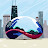Joseph Rayner says:

if you put a dislike take it off this man spent 4 hours helping us

13.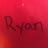Ryan says:

22:43 You made a mistake by saying "17 over 5", when you meant to say "17 over 15"

14.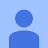Anonymous says:

1:35:00

15.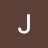Jorge Hernandezpacheco says:

Fbhgyysvxtd sie

16.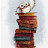After all this time? Always says:

Anyone else have Algebra 2 spring semester last year and now you're trying to learn all of algebra 2 by yourself before taking the SAT 💀

17.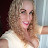Denise Dattilo says:

Again you repeated the same error, this is timestamp -244:30 where you didn't remove the negative sign when placing it on the other side of the equal sign which makes this equation incorrect 😳

18.Denise Dattilo says:

In your example timed stamped -253:13
You forgot to drop off the negative sign when switching to the other side of the equal sign, which made the equation incorrect just saying 😏

19.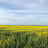Raymond Z says:

Guy: Alr, time for some fun and easy math basics!!

10 min in: Alr, are we done?

30min in: Bruh

1h: GHEJFUWILJI

2H; Playing valorant and games and switching tabs once mom comes close

3h; Gets so complicated that you just fuk up

4h: Thers no 4h GODIE

20.Ahmad Rafa says:

Algebra 2: electric boogaloo

21.simplyella says:

ty studying for my damn final

22.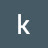krish patel says:

ALL TIMESTAMPS {Compiled} THIS IS A COPY TO KEEP IT IN MY PLACE, YOU CAN USE IT OR YOU CAN IGNORE THANKS

0:00 | Intro

~~LINEAR EQUATIONS~~

0:07 | Basic

1:03 | Variables on Both Sides

2:24 | Parentheses/Distribution

5:00 | Fraction Coefficients

6:06 | Fraction Coefficients and Constants

7:39 | Fractions on Both Sides (Cross Multiplication)

~~INEQUALITIES~~

8:31 | Basic Graphing

10:36 | Interval Notation

11:33 | Inequality Equations

13:45 | OR Inequalities

15:53 | AND Inequalities

~~ABSOLUTE VALUE~~

17:40 | Basic

18:17 | Equations

20:30 | Equations with Numbers Outside

22:50 | Absolute Value Inequalities

26:38 | Inequalities with Numbers Outside

~~GRAPHING~~

30:04 | Basic Slope-Intercept

31:54 | Fraction Slope

33:07 | Standard Form

35:21 | Linear Inequalities

41:48 | Absolute Value Functions

49:50 | Absolute Value Inequalities

53:04 | Constants

~~FINDING SLOPE AND WRITING EQUATIONS~~

53:46 | Slope Between 2 Points

56:00 | Slope Between 2 Points with Fractions

59:19 | Ways to Write an Equation, Point-Slope Form Intro

1:00:20 | Writing Equations in Standard and Slope-Intercept Form

1:02:49 | Writing Equations in Point Slope, Slope-Intercept, and Standard Form

1:06:29 | When Given 2 Points

1:11:39 | Using Line Relationships and a Point

1:15:16 | Rate of Change Word Problem

~~EXPONENTS~~

1:17:22 | Exponent Rules

~~SYSTEMS~~

1:23:26 | Systems of Equations

1:30:40 | Graphing

1:33:10 | Difference of Squares

1:36:00 | Factoring with GCF

1:41:36 | Quadratic Formula and Discriminant

1:56:21 | Completing the Square

2:03:02 | Graphing

2:14:25 | Graphing from Standard Form, finding zeroes/roots/x intercepts

~~DOMAIN AND RANGE~~

2:20:03 | Domain and Range of Linear and Absolute Value Functions

~~IMAGINARY NUMBERS~~

2:23:17 | Imaginary Number Fractions

~~MORE DOMAIN AND RANGE~~

2:24:47 | Domain and Range of Absolute Value Functions

2:25:15 | Domain and Range of Quadratics

~~MORE IMAGINARY NUMBERS~~

2:26:16 | Intro to Imaginary Numbers

2:27:57 | Operations

2:33:10 | Graphing

2:35:30 | Exponents on Imaginary Numbers

2:38:35 | Finding Zeroes

2:40:43 | Rational Zero Test and Synthetic Division~~Functions~~

2:48:40 | Calculating functions by plugging x values

2:50:00 | Calculating functions using synthetic division

~~POLYNOMIALS~~

2:51:30 | Dividing expressions using synthetic division

2:53:00 | Dividing expressions using long division

~~Composite Functions~~

2:55:45 | One function inside another. ex. f(g(x))

~~INVERSE FUNCTIONS~~

2:59:00 | Finding f^-1(x)

3:02:05 | Proving that two functions are inverse

~~LOGARITHMS~~

3:04:30 | Finding logs

3:15:05 | Logs with variable x

3:17:20 | Adding/ Subtracting logs with variable x

3:26:43 | Rationalizing expressions

3:30:40 | Solving radical expressions with variable x

~~RATIONAL EXPRESSIONS (FRACTIONS)~~

3:35:52 | Multiplying/Dividing

3:45:06 | Solving for x

3:50:45 | Graphing rational expressions

23.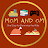Mom And Om says:

Literally spent 4 hours to lecture algebra 2… round of applause.. was able to understand everything even as a 3rd grader

24.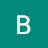Blake Castleberg says:

I love how I'm about to take my algebra 2 final yet I'm watching an intro to algebra 2. I need to get out of online school or something…fuck

25.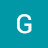German Rojas says:

So much shit yo

26.Simply Amber says:

algebra,
your x ain't comin' back so consider finding a new one

27.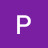PurpleZinnia says:

Why does the education make math teaching so difficult? The Organic Chemistry Tutor is not a teacher in a good way. He explains and shows how to solve problems while respecting one's intelligence and doesn't shame one for not understanding or makes one feel like a dummy and unworthy. (Yeah, I had issues in math class.) (smile emoji) I had a math tutor who never trained as a math teacher and he was the best "math teacher" I ever had. The Organic Chemistry Tutor is the another best "math teacher".

28.PurpleZinnia says:

Thanks for the videos. I wish my classes had been this engaging. Why your videos rock–you use math terms but explain how to solve a problem in plain language. The graphics make it easy to follow along. You're passionate about math but speak calmly and deliberately. You recognize the viewer's intelligence and don't talk down to them. After watching the video, I felt smart, capable and confident. I'm starting to "like" math.

29.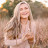Tate says:

turn down the video speed if you need to!!! i completely for got about that:) so if it’s to fast for you then do that!!

30.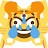The Imperial says:

ALL TIMESTAMPS {Compiled}

0:00 | Intro

~~LINEAR EQUATIONS~~

0:07 | Basic

1:03 | Variables on Both Sides

2:24 | Parentheses/Distribution

5:00 | Fraction Coefficients

6:06 | Fraction Coefficients and Constants

7:39 | Fractions on Both Sides (Cross Multiplication)

~~INEQUALITIES~~

8:31 | Basic Graphing

10:36 | Interval Notation

11:33 | Inequality Equations

13:45 | OR Inequalities

15:53 | AND Inequalities

~~ABSOLUTE VALUE~~

17:40 | Basic

18:17 | Equations

20:30 | Equations with Numbers Outside

22:50 | Absolute Value Inequalities

26:38 | Inequalities with Numbers Outside

~~GRAPHING~~

30:04 | Basic Slope-Intercept

31:54 | Fraction Slope

33:07 | Standard Form

35:21 | Linear Inequalities

41:48 | Absolute Value Functions

49:50 | Absolute Value Inequalities

53:04 | Constants

~~FINDING SLOPE AND WRITING EQUATIONS~~

53:46 | Slope Between 2 Points

56:00 | Slope Between 2 Points with Fractions

59:19 | Ways to Write an Equation, Point-Slope Form Intro

1:00:20 | Writing Equations in Standard and Slope-Intercept Form

1:02:49 | Writing Equations in Point Slope, Slope-Intercept, and Standard Form

1:06:29 | When Given 2 Points

1:11:39 | Using Line Relationships and a Point

1:15:16 | Rate of Change Word Problem

~~EXPONENTS~~

1:17:22 | Exponent Rules

~~SYSTEMS~~

1:23:26 | Systems of Equations

1:30:40 | Graphing

1:33:10 | Difference of Squares

1:36:00 | Factoring with GCF

1:41:36 | Quadratic Formula and Discriminant

1:56:21 | Completing the Square

2:03:02 | Graphing

2:14:25 | Graphing from Standard Form, finding zeroes/roots/x intercepts

~~DOMAIN AND RANGE~~

2:20:03 | Domain and Range of Linear and Absolute Value Functions

~~IMAGINARY NUMBERS~~

2:23:17 | Imaginary Number Fractions

~~MORE DOMAIN AND RANGE~~

2:24:47 | Domain and Range of Absolute Value Functions

2:25:15 | Domain and Range of Quadratics

~~MORE IMAGINARY NUMBERS~~

2:26:16 | Intro to Imaginary Numbers

2:27:57 | Operations

2:33:10 | Graphing

2:35:30 | Exponents on Imaginary Numbers

2:38:35 | Finding Zeroes

2:40:43 | Rational Zero Test and Synthetic Division~~Functions~~

2:48:40 | Calculating functions by plugging x values

2:50:00 | Calculating functions using synthetic division

~~POLYNOMIALS~~

2:51:30 | Dividing expressions using synthetic division

2:53:00 | Dividing expressions using long division

~~Composite Functions~~

2:55:45 | One function inside another. ex. f(g(x))

~~INVERSE FUNCTIONS~~

2:59:00 | Finding f^-1(x)

3:02:05 | Proving that two functions are inverse

~~LOGARITHMS~~

3:04:30 | Finding logs

3:15:05 | Logs with variable x

3:17:20 | Adding/ Subtracting logs with variable x

3:26:43 | Rationalizing expressions

3:30:40 | Solving radical expressions with variable x

~~RATIONAL EXPRESSIONS (FRACTIONS)~~

3:35:52 | Multiplying/Dividing

3:45:06 | Solving for x

3:50:45 | Graphing rational expressions

31.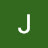JosephNathan says:

I watch all it

32.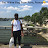Antwan Wimberly says:

yall remember the one fine European chick who said she hates guys w/ grey hair – she wound up dead af – show some love for the veterans mang – colors

33.Antwan Wimberly says:

vKewllll I went home to Paris and France and Europe years ago – my bags are truly from overseas in this photo – France won af post integration – well – sort of – it's still ours af – #worldcup asf young – we do sports in our leisure time and spare time af – #stem af – me and bill gates need to make up for all that lost time studying we should both be 6,8 inches tall af

34.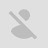Darshan Patel says:

I have a EOC next week, and I'm hoping this whole review will help me with it!

35.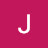J Chr G says:

Let's go back to the fun parts, because solving (solving anything) means first and foremost that you need to understand what you are looking at or reading, or put it like this way you need to be able to shape it out of numbers first to put it in bits of understandable grammar(/text) and vise versa
I never approach to get the answer immediately
Approach methods may differ from individual to individual.
Mathematics/algebra and physics go hand in hand
You need to understand it in nature as well as the answers should be recognisable and corresponding to natures laws

36.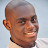Quabeenuh says:

👋

37.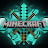JandF GAMING says:

this actualy realy helped me out allot as i have allot of school work on algebra that im not very good at you are amazing

38.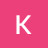Kevin Orr says:

I’m 56. I just subscribed simply to support you for your effort.

39.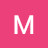Martha Grevol says:

Im going through matric and I feel with almost high hopes that I might do well in maths because of you. You are amazing,, i want to recommend you to all my friends.

40.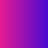Kyle Sul says:

Everyone is here to learn and get smarter mean while I'm here just trying to sleep

41.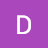Dr. Hamath MD. says:

I learned arithmetic in school the foundation of all other " MATHEMATICS".

42.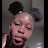Ta'Briyah Johnson says:

43.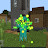Tony Tello says:

Here because learning online is hard

44.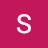StreetArtillery says:

4:55 x=-6/5 is an improper fraction. Correct answer is -1 1/5 or -1.2

45.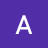Angelo Mrsic says:

when you skip one day of class and everyone else learns the whole of algebra 2

46.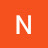Nathaniel Thorne says:

Thank you.

47.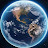Hermanosponce9 says:

1:16:45 “ the ROBBIT population” lmao

48.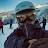Jiwen Yang says:

wow…